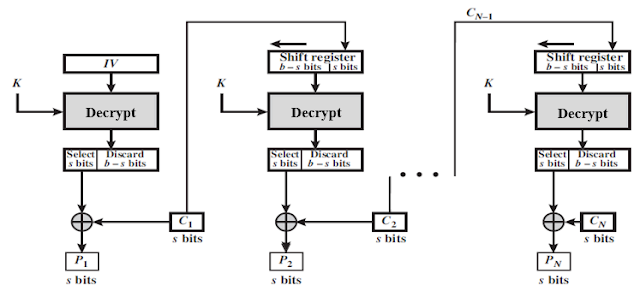## Sunday, October 17, 2021

### CFB Mode | Cipher Feedback Mode | Mode of Block Cipher | Application of CFB Mode

Introduction

Cryptographic algorithm works on main two techniques: block and stream ciphers.

In a stream cipher, the plaintext is encrypted one bit at a time. In a block cipher, the plaintext is broken into blocks of a fixed length and the bits in each block are encrypted together. One of the main issues with block ciphers is that they only allow you to encrypt messages the fixed size as their block length.

If plaintext, which has a block size 64 bits easily encrypt. But encrypt a 65-bit message, you need a way to define how the second block should be encrypted.

The solution to this is called block cipher modes of operation. Need of block cipher mode is basic building block for providing data security. In block cipher rather than encrypting one bit at a time, block of bits is encrypted at a time.

There are 5 modes of operation for block cipher that may be used in a wide variety of applications like symmetric key cryptographic algorithm. These modes define how data encrypted and decrypted.

Cipher Feedback Mode (CFB)

CFB mode use block cipher, but act as stream cipher. It means data is encrypted in smaller units of block 8-bits rather than predefined size of 64-bits. In CFB encryption process 64 bits initialization vector is used which kept in 64 bits of shift register. The Initial Vector (IV) is encrypted and produces 64-bits encrypted IV. It is divided in to two parts s(8-bits) and b-s (remaining 56 bits).

Now the leftmost s bits (size of 8 bits) of the encrypted IV are XORed with the first s bits (size of 8 bits) of plain text P1, to produce the first s bits of cipher text C1, which is then transmitted to next step. In next step content of the 64-bit shift register are shifted left by b-s bits and C1 is placed in the right most s bits of the shift register and which again undergoes to encryption process shown in figure. This process continue until all plain text units have been encrypted.Figure: Encryption Process of CFB Mode

In decryption, same process performs shown in figure. Here same key K is used during encryption and decryption process. CFB mode is converting a block cipher into a type of stream cipher.Figure: Decryption Process of CFB Mode

Application of CFB Mode

This algorithm mostly used to key stream generator to produce key stream.